# python中plot实现即时数据动态显示方法

`本人同类型博客(新鲜的哦！)`matplotlib animation 绘制动画： 数据收集（产生）完成后，再生成动态显示。一般用于成果展示。生成各种格式的视频,gif动态图等。

Matlab使用Plot函数实现数据动态显示方法总结中介绍了两种实现即时数据动态显示的方法。考虑到使用python的人群日益增多，再加上本人最近想使用python动态显示即时的数据，网上方法很少，故总结于此。本人主要在jupyter notebook作为交互式的python运行环境，但考虑不使用jupyter notebook的情况。所以下面主要分成针对jupyter notebook环境的增量式数据动态显示方法，与通用的（jupyter notebook 与非juypter notebook都适用）方法两大类。先介绍通用的方法，后介绍针对jupyter notebook的方法。接着给出一个更加实用的简单例子，希望可以给大家更多的启发。最后对博友运行本程序时遇到的问题做一个总结，并给出解决方法。

## 1. 通用的方法

### 1.1 需要保存历史数据

``````import matplotlib.pyplot as plt
import numpy as np
import time
from math import *

plt.ion() #开启interactive mode 成功的关键函数
plt.figure(1)
t = 
t_now = 0
m = [sin(t_now)]

for i in range(2000):
plt.clf() #清空画布上的所有内容
t_now = i*0.1
t.append(t_now)#模拟数据增量流入，保存历史数据
m.append(sin(t_now))#模拟数据增量流入，保存历史数据
plt.plot(t,m,'-r')
plt.draw()#注意此函数需要调用
time.sleep(0.01)

`备注：此代码经过很多人测试发现不能呈现动态效果（我原本在ubuntu系统上是可以的，经验证在windows上也不行），特在此说明，如果出不了动态效果，就将`

``````plt.draw()
time.sleep(0.01)

`替换为:`

``````plt.pause(0.01)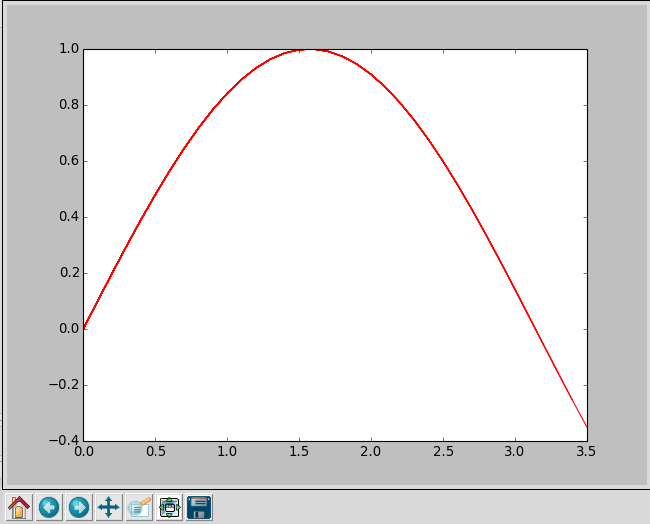### 1.2 无需保存数据

``````import matplotlib.pyplot as plt
import numpy as np
import time
from math import *

plt.ion() #开启interactive mode 成功的关键函数
plt.figure(1)
t = 
t_now = 0
m = [sin(t_now)]

for i in range(2000):
# plt.clf() # 清空画布上的所有内容。此处不能调用此函数，不然之前画出的点，将会被清空。
t_now = i*0.1
"""
由于第次只画一个点，所以此处有两种方式，第一种plot函数中的样式选
为点'.'、'o'、'*'都可以，就是不能为线段'-'。因为一条线段需要两
个点才能确定。第二种方法是scatter函数，也即画点。
"""
plt.plot(t_now,sin(t_now),'.') # 第次对画布添加一个点，覆盖式的。
# plt.scatter(t_now, sin(t_now))

plt.draw()#注意此函数需要调用
time.sleep(0.01)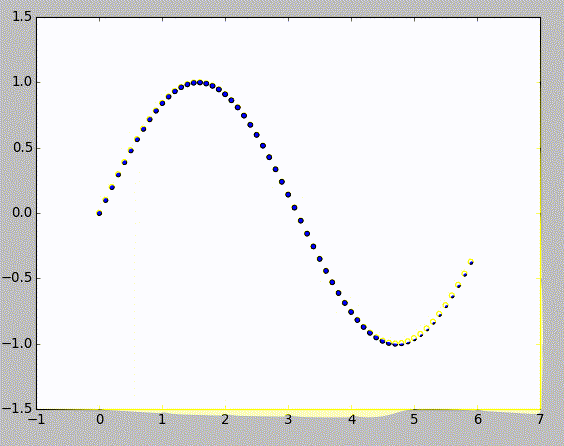### 1.3 无需保存数据（进阶版）

``````import matplotlib.pyplot as plt
import numpy as np
import time
from math import *

plt.ion() #开启interactive mode 成功的关键函数
plt.figure(1)
t = np.linspace(0, 20, 100)

for i in range(20):
# plt.clf() # 清空画布上的所有内容。此处不能调用此函数，不然之前画出的轨迹，将会被清空。
y = np.sin(t*i/10.0)
plt.plot(t, y) # 一条轨迹
plt.draw()#注意此函数需要调用
time.sleep(1)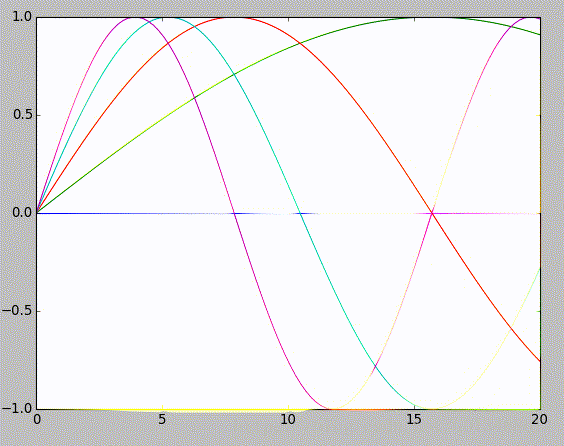## 2. Jupyter notebook版本

### 2.1 需要保存历史数据

``````import math
import random
import numpy as np
import matplotlib
import matplotlib.pyplot as plt
%matplotlib inline

# set up matplotlib
is_ipython = 'inline' in matplotlib.get_backend()
if is_ipython:
from IPython import display

plt.ion()

def plot_durations(y):
plt.figure(2)
plt.clf()
plt.subplot(211)
plt.plot(y[:,0])
plt.subplot(212)
plt.plot(y[:,1])

plt.pause(0.001)  # pause a bit so that plots are updated
if is_ipython:
display.clear_output(wait=True)
display.display(plt.gcf())

x = np.linspace(-10,10,500)
y = []
for i in range(len(x)):
y1 = np.cos(i/(3*3.14))
y2 = np.sin(i/(3*3.14))
y.append(np.array([y1,y2])) #保存历史数据
plot_durations(np.array(y))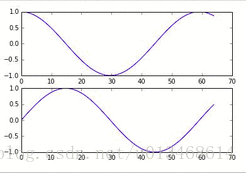### 2.2 无需保存历史数据

``````import math
import random
import numpy as np
import matplotlib
import matplotlib.pyplot as plt
%matplotlib inline

# set up matplotlib
is_ipython = 'inline' in matplotlib.get_backend()
if is_ipython:
from IPython import display

plt.ion()

def plot_durations(i, y1, y2):
plt.figure(2)
#     plt.clf() 此时不能调用此函数，不然之前的点将被清空。
plt.subplot(211)
plt.plot(i, y1, '.')
plt.subplot(212)
plt.plot(i, y2, '.')

plt.pause(0.001)  # pause a bit so that plots are updated
if is_ipython:
display.clear_output(wait=True)
display.display(plt.gcf())

x = np.linspace(-10,10,500)
y = []
for i in range(len(x)):
y1 = np.cos(i/(3*3.14))
y2 = np.sin(i/(3*3.14))
#     y.append(np.array([y1,y2])) #保存历史数据
plot_durations(i, y1, y2)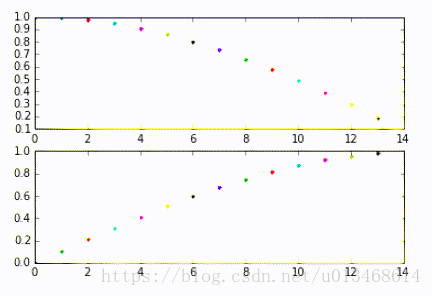### 2.3 无需保存历史数据（进阶版）

``````import math
import random
import numpy as np
import matplotlib
import matplotlib.pyplot as plt
%matplotlib inline

# set up matplotlib
is_ipython = 'inline' in matplotlib.get_backend()
if is_ipython:
from IPython import display

plt.ion()

def plot_durations(y1, y2):
plt.figure(2)
#     plt.clf()
plt.subplot(211)
plt.plot(x, y1)
plt.subplot(212)
plt.plot(x, y2)

plt.pause(0.001)  # pause a bit so that plots are updated
if is_ipython:
display.clear_output(wait=True)
display.display(plt.gcf())

x = np.linspace(-10,10,500)
for i in range(100):
y1 = np.cos(x*i/(3*3.14))
y2 = np.sin(x*i/(3*3.14))
plot_durations(y1, y2)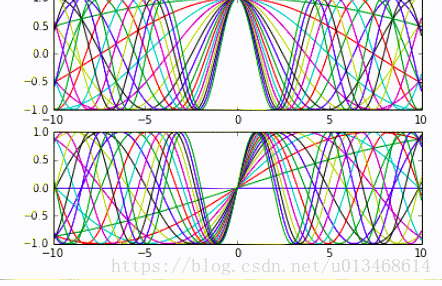## 4. 问题与解决

1. `plt.draw() time.sleep(0.01)`替换为`plt.pause(0.01)`运行，看是否正常。
2. 如果还不行，就把`plt.pause(0.01)`也注释掉再运行。